# Examples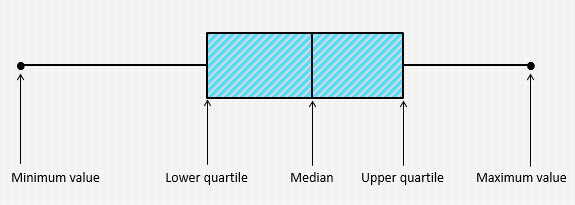A box plot may show key statistics such as the median of the data, maximum and minimum values, as well as the lower and upper quartiles.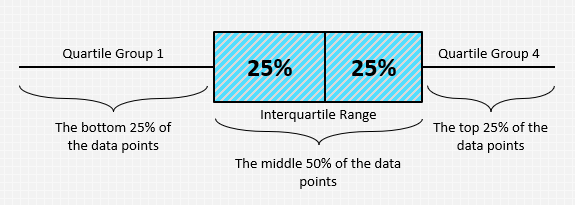The data is plotted in a box plot in such away that the bottom 25% and the top 25% of the data points are represented by the two whiskers, whereas the middle 50% of the data points are represented by the box.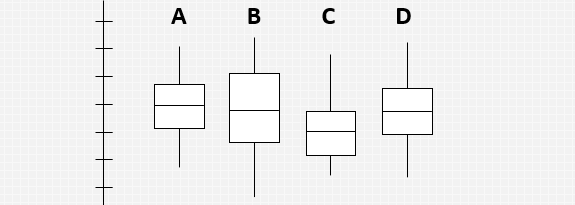Box plots are most useful when comparing between several data sets. They allow you to compare the central tendency as well as the variability of multiple data sets.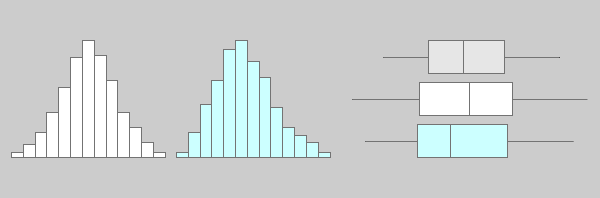Box plots are most useful when comparing between several data sets. They are less detailed than histograms, and take up less space which make them easier to compare.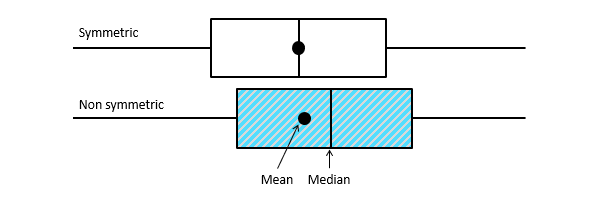Box plots can tell if the distribution is symmetrical or skewed. In a symmetric distribution, the mean and median are nearly the same, and the two whiskers has almost the same length.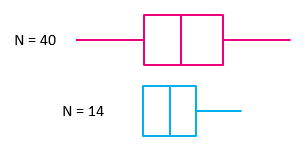Box plots are ideal to represent moderate to large amount of data. The size of the box plot can vary significantly if the data size is small.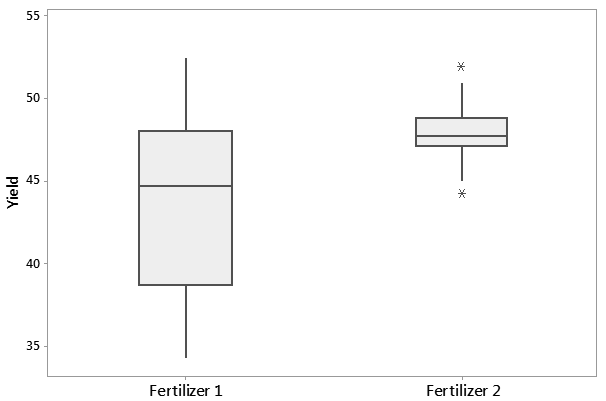The following are boxplots that display the yield of a crop after applying two different fertilizers. Fertilizer 2 appears to have a higher yield than Fertilizer 1.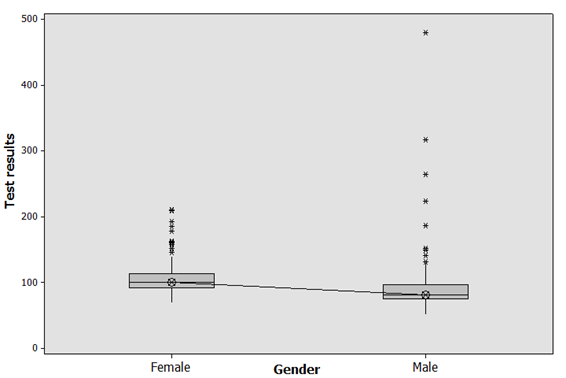This example shows that females have higher glucose levels than males in a workplace. ANOVA can be used here to test the significance of the difference between the two means.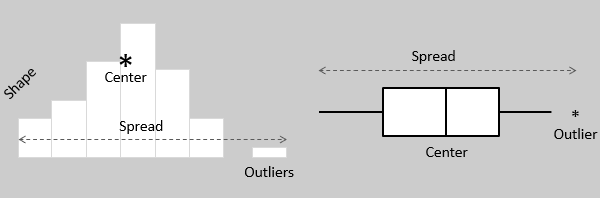Both histograms and boxplots allow to visually assess the central tendency, the amount of variation in the data as well as the presence of gaps, outliers or unusual data points.